# Re-analysis of an Agreement Study

library(SimplyAgree)
#library(tidyverse)
library(dplyr)
library(tidyr)
library(ggplot2)
library(magrittr)
data("temps")
df_temps = temps

# Re-analysis of a Previous Study of Agreement

In the study by Ravanelli and Jay (2020), they attempted to estimate the effect of varying the time of day (AM or PM) on the measurement of thermoregulatory variables (e.g., rectal and esophageal temperature). In total, participants completed 6 separate trials wherein these variables were measured. While this is a robust study of these variables the analyses focused on ANOVAs and t-tests to determine whether or not the time-of-day (AM or PM). This poses a problem because 1) they were trying to test for equivalence and 2) this is a study of agreement not differences (See Lin (1989)). Due to the latter point, the use of t-test or ANOVAs (F-tests) is rather inappropriate since they provide an answer to different, albeit related, question.

Instead, the authors could test their hypotheses by using tools that estimate the absolute agreement between the AM and PM sessions within each condition. This is rather complicated because we have multiple measurement within each participant. However, between the tools included in SimplyAgree1 I believe we can get closer to the right answer.

In order to understand the underlying processes of these functions and procedures I highly recommend reading the statistical literature that documents methods within these functions. For the cccrm package please see the work by Josep L. Carrasco and Jover (2003), Josep L. Carrasco, King, and Chinchilli (2009), and Josep L. Carrasco et al. (2013). The loa_lme function was inspired by the work of Parker et al. (2016) which documented how to implement multi-level models and bootstrapping to estimate the limits of agreement.

# Concordance

An easy approach to measuring agreement between 2 conditions or measurement tools is through the concordance correlation coefficient (CCC). The CCC essentially provides a single coefficient (values between 0 and 1) that provides an estimate to how closely one measurement is to another. It is a type of intraclass correlation coefficient that takes into account the mean difference between two measurements. In other words, if we were to draw a line of identity on a graph and plot two measurements (X & Y), the closer those points are to the line of identity the higher the CCC (and vice versa).

qplot(1,1) + geom_abline(intercept = 0, slope = 1)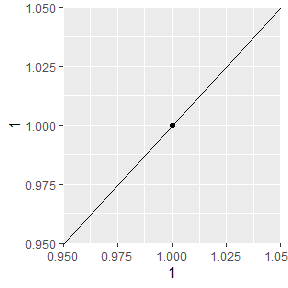Example of the Line of Identity

In the following sections, let us see how well esophageal and rectal temperature are in agreement after exercising in the heat for 1 hour at differing conditions.

## Rectal Temperature

We can visualize the concordance between the two different types of measurements and the respective time-of-day and conditions. From the plot we can see there is clear bias in the raw post exercise values (higher in the PM), but even when “correcting for baseline differences” by calculating the differences scores we can see a higher degree of disagreement between the two conditions.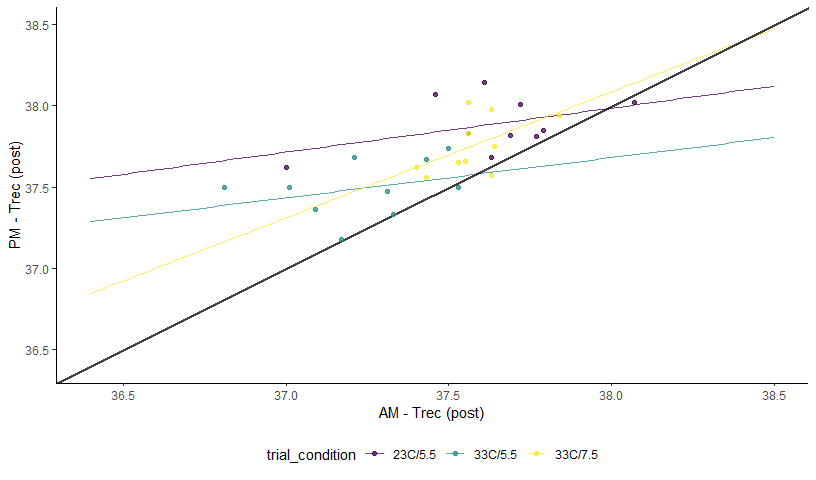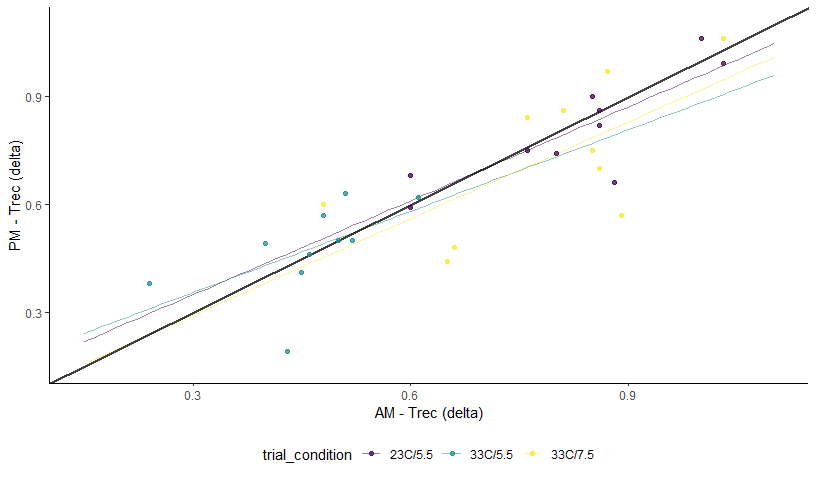# Esophageal Temperature

#> Warning: Removed 3 row(s) containing missing values (geom_path).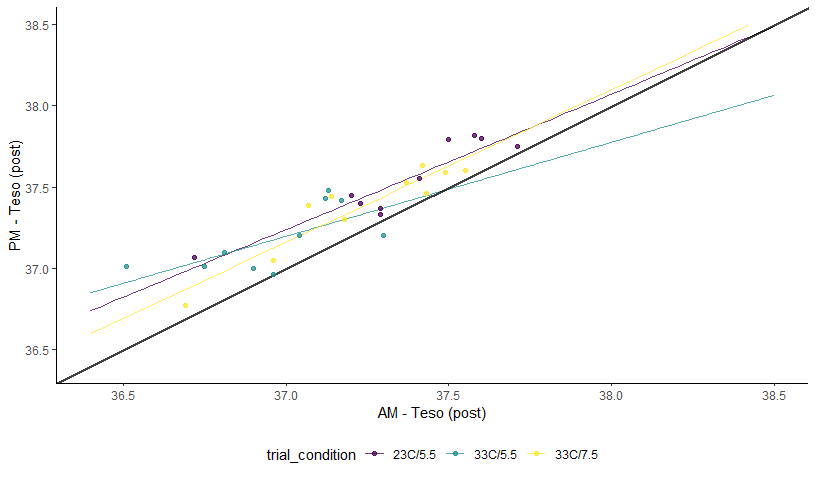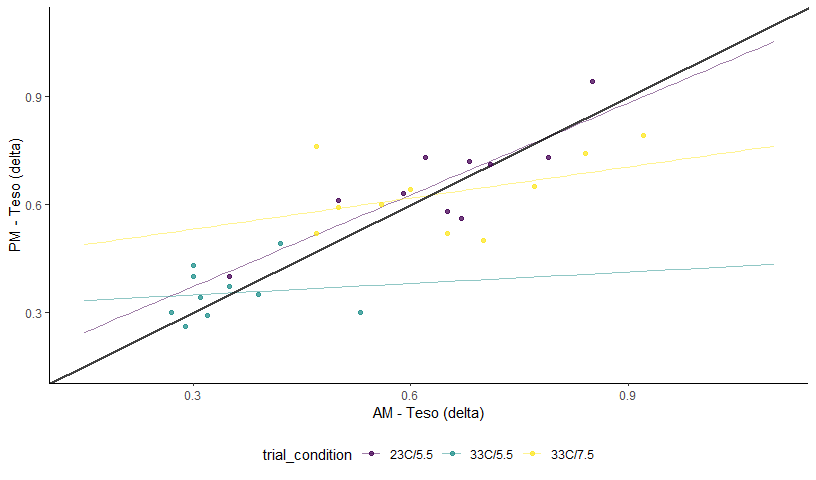# Limits of Agreement

The loa_lme function can be used to calculate the “limits of agreement”. Typically the 95% Limits of Agreement are calculated which provide the difference between two measuring systems for 95% of future measurements pairs. In order to do that we will need the data in a “wide” format where each measurement (in this case AM and PM) are their own column and then we can calculate a column that is the difference score. Once we have the data in this “wide” format, we can then use the loa_lme function to calculate the average difference (mean bias) and the variance (which determines the limits of agreement).

## Rectal Temperature

So we will calculate the limits of agreement using the loa_lme function. We will need to identify the columns with the right information using the diff, avg, condition, and id arguments. We then select the right data set using the data argument. Lastly, we specify the specifics of the conditions for how the limits are calculated. For this specific analysis I decided to calculate 95% limits of agreement with 95% confidence intervals, and I will use percentile bootstrap confidence intervals.

rec.post_loa = SimplyAgree::loa_lme(diff = "diff",
condition = "trial_condition",
id = "id",
avg = "Average",
data = df_rec.post,
conf.level = .95,
agree.level = .95,
replicates = 199,
type = "perc")

When we create a table of the limits of agreement (LoA), at least for Trec post exercise, are providing the same conclusion (poor agreement).

knitr::kable(rec.post_loa$loa, caption = "LoA: Trec Post Exercise") LoA: Trec Post Exercise term condition estimate bias se lower.ci upper.ci Bias 23C/5.5 0.2550000 -0.0114061 0.0707957 0.1058965 0.3750531 Bias 33C/5.5 0.2540000 0.0044150 0.0724833 0.1071222 0.4044288 Bias 33C/7.5 0.1810000 -0.0084558 0.0708715 0.0263866 0.3118697 Lower LoA 23C/5.5 -0.1708766 -0.0129563 0.0919009 -0.3746394 0.0005661 Lower LoA 33C/5.5 -0.1718766 0.0028648 0.0943708 -0.3478418 0.0107792 Lower LoA 33C/7.5 -0.2448766 -0.0100059 0.0875189 -0.4389267 -0.0866145 Upper LoA 23C/5.5 0.6808766 -0.0098559 0.0911554 0.4887056 0.8675360 Upper LoA 33C/5.5 0.6798766 0.0059652 0.0912843 0.5129644 0.8784498 Upper LoA 33C/7.5 0.6068766 -0.0069056 0.0954830 0.3783011 0.7849023 Furthermore, we can visualize the results with a typical Bland-Altman plot of the LoA. plot(rec.post_loa)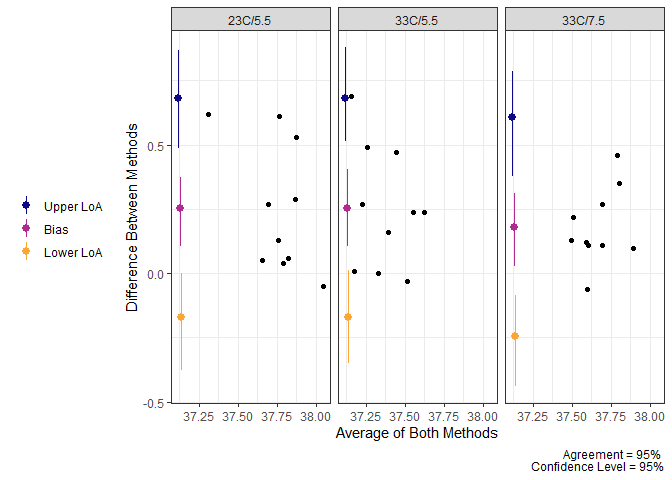Limits of Agreement for Trec Post Exercise Now, when we look at the Delta values for Trec we find that there is much closer agreement (maybe even acceptable agreement) when we look at LoA. However, we cannot say that the average difference would be less than 0.25 which may not be acceptable for some researchers. knitr::kable(rec.delta_loa$loa,
caption = "LoA: Delta Trec")
LoA: Delta Trec
term condition estimate bias se lower.ci upper.ci
Bias 23C/5.5 -0.0190000 -0.0011451 0.0396377 -0.0949281 0.0630221
Bias 33C/5.5 0.0150000 -0.0014430 0.0372452 -0.0497428 0.0910483
Bias 33C/7.5 -0.0590000 0.0000772 0.0391803 -0.1331558 0.0159793
Lower LoA 23C/5.5 -0.2532956 0.0015001 0.0533380 -0.3570648 -0.1638991
Lower LoA 33C/5.5 -0.2192956 0.0012022 0.0479123 -0.3048820 -0.1172316
Lower LoA 33C/7.5 -0.2932956 0.0027224 0.0534793 -0.3946780 -0.1885677
Upper LoA 23C/5.5 0.2152956 -0.0037903 0.0510084 0.1028572 0.3286267
Upper LoA 33C/5.5 0.2492956 -0.0040881 0.0527573 0.1473752 0.3507382
Upper LoA 33C/7.5 0.1752956 -0.0025680 0.0501465 0.0733961 0.2711665
plot(rec.delta_loa)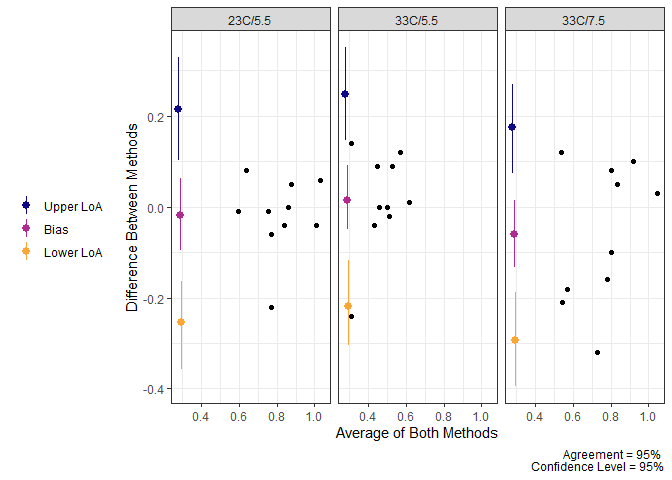Limits of Agreement for Delta Trec

## Esophageal Temperature

We can repeat the process for esophageal temperature. Overall, the results are fairly similar, and while there is better agreement on the delta (change scores), it is still fairly difficult to determine that there is “good” agreement between the AM and PM measurements.

eso.post_loa = SimplyAgree::loa_mixed(diff = "diff",
condition = "trial_condition",
id = "id",
data = df_eso.post,
conf.level = .95,
agree.level = .95,
replicates = 199,
type = "bca")
knitr::kable(eso.post_loa$loa, caption = "LoA: Teso Post Exercise") LoA: Teso Post Exercise term condition estimate bias se lower.ci upper.ci Bias 23C/5.5 0.180000 0.0054383 0.0414297 0.1018153 0.2727195 Bias 33C/5.5 0.212000 0.0003411 0.0409850 0.1225349 0.2781630 Bias 33C/7.5 0.146000 0.0067433 0.0411734 0.0699717 0.2437454 Lower LoA 23C/5.5 -0.078867 0.0087499 0.0577083 -0.1882852 0.0362440 Lower LoA 33C/5.5 -0.046867 0.0036528 0.0562961 -0.1450997 0.0710961 Lower LoA 33C/7.5 -0.112867 0.0100549 0.0533235 -0.2205322 0.0096297 Upper LoA 23C/5.5 0.438867 0.0021267 0.0519004 0.3284062 0.5399114 Upper LoA 33C/5.5 0.470867 -0.0029705 0.0527384 0.3570791 0.5748910 Upper LoA 33C/7.5 0.404867 0.0034317 0.0560191 0.3054799 0.5188644 plot(eso.post_loa)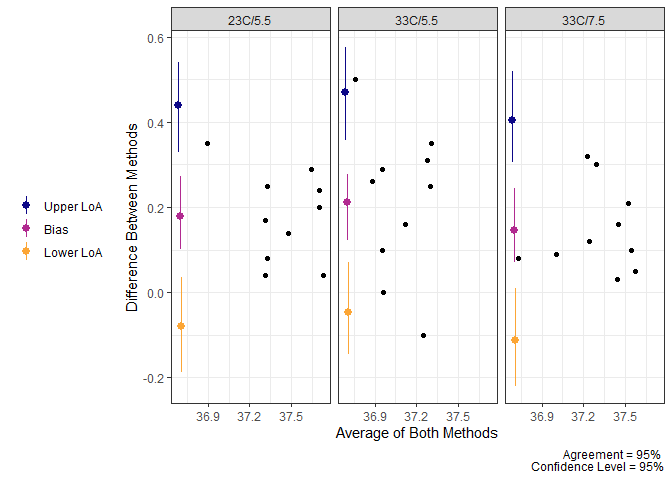Limits of Agreement for Teso Post Exercise knitr::kable(eso.delta_loa$loa,
caption = "LoA: Delta Teso")
LoA: Delta Teso
term condition estimate bias se lower.ci upper.ci
Bias 23C/5.5 0.0200000 -0.0032266 0.0346942 -0.0528344 0.0930582
Bias 33C/5.5 0.0050000 -0.0009160 0.0346594 -0.0687195 0.0839039
Bias 33C/7.5 -0.0170000 -0.0026115 0.0345165 -0.0870805 0.0516728
Lower LoA 23C/5.5 -0.1992475 -0.0007217 0.0467556 -0.2940191 -0.1094022
Lower LoA 33C/5.5 -0.2142475 0.0015889 0.0452052 -0.2997399 -0.1297578
Lower LoA 33C/7.5 -0.2362475 -0.0001066 0.0480083 -0.3370631 -0.1477120
Upper LoA 23C/5.5 0.2392475 -0.0057314 0.0474541 0.1312817 0.3429716
Upper LoA 33C/5.5 0.2242475 -0.0034208 0.0488839 0.1350650 0.3297975
Upper LoA 33C/7.5 0.2022475 -0.0051163 0.0459192 0.1140479 0.2865703
plot(eso.delta_loa)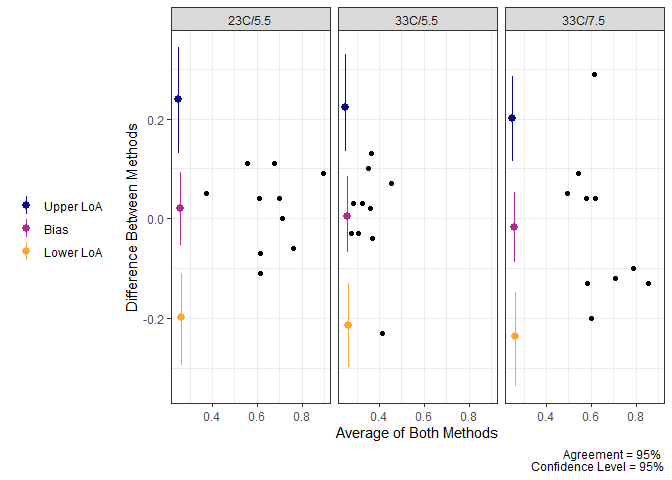Limits of Agreement for Delta Teso

1. Josep Lluis Carrasco and Martinez (2020) is another package to check out↩︎